# Test: Gaseous State - 4

## 20 Questions MCQ Test Physical Chemistry | Test: Gaseous State - 4

Description
This mock test of Test: Gaseous State - 4 for Chemistry helps you for every Chemistry entrance exam. This contains 20 Multiple Choice Questions for Chemistry Test: Gaseous State - 4 (mcq) to study with solutions a complete question bank. The solved questions answers in this Test: Gaseous State - 4 quiz give you a good mix of easy questions and tough questions. Chemistry students definitely take this Test: Gaseous State - 4 exercise for a better result in the exam. You can find other Test: Gaseous State - 4 extra questions, long questions & short questions for Chemistry on EduRev as well by searching above.
QUESTION: 1

### Maximum number of electrons in a subshell with l = 3 and n = 4 is

Solution:

n = 4, l  =3

In f subshell, there are 7 orbitals and each orbital can accommodate a maximum of two electrons, so, maximum no. of electrons in 4f subshell = 7×2 = 14

QUESTION: 2

Solution:
QUESTION: 3

### The electronic configuration of a dipositive metal M2+ is 2, 8, 14 and its atomic weight is 56 a.m.u. The number of neutrons in its nuclei would be

Solution: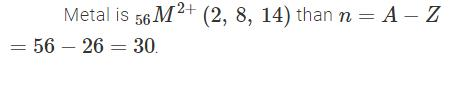QUESTION: 4

Which in not correct curve for gay-lussac’s law:

Solution:
QUESTION: 5

Which is incorrect curve for Boyle’s law:

Solution:
QUESTION: 6

The energy of an electron in first Bohr orbit of H-atom is –13.6 eV. The possible energy value of electron in the excited state of Li2+ is

Solution: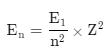where

E1​=Energy of first Bohr orbit =−13.6eV

n= no of orbit

Z=Atomic no

For Li2+, the excited state, n=2 and Z=3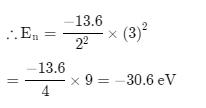QUESTION: 7

Which of the following curve does not represent gay lusacc’s law:

Solution:

P = KT
P = K(tC+273)

QUESTION: 8

For the gaseous reaction, the rate is often expressed in terms of dp/dt instead of dC/dt or dn/dt. What is the relationship among these three expression:

Solution:
QUESTION: 9

A gas can be liquefied most suitably at:

Solution:

For liquefaction of gas temperature should be lower than critical temperature. Also higher is the P in comparison to critical pressure easier is liquefaction .

QUESTION: 10

For a closed (not rigid) container containing n = 10 moles of an ideal gas fitted with movable, frictionless, weightless piston operating such that pressure of gas remains constant at 0.821 atm, which graph represents correct variation of log V vs log T where V is in litre and T in Kelvin:

Solution:
QUESTION: 11

At a definite temperature (T), the distribution of velocities is given by the curve. The curve that indicates that the velocity corresponding to points A, B and C are: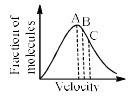Solution:
QUESTION: 12

A graph is plotted between P (atm) vs t°C for 10 mol of an ideal gas as follows: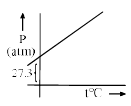Then slope of curve and volume of container (L) respectively, is

Solution:
QUESTION: 13

For two samples A and B of ideal gas following curve is plotted between n vs V (volume) of container) at 16.42 atm pressure as follows, then temperature of A and B respectively are: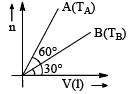Solution:
QUESTION: 14

Match the Column-I and Column-II: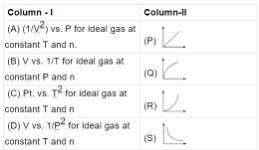Solution:

Explanation : A-Q, B-S, C-P, D-R

QUESTION: 15

Match the correct from List-1 to List-2 on the basic of following Andrews isotherm of Real gas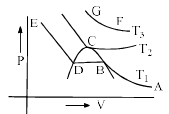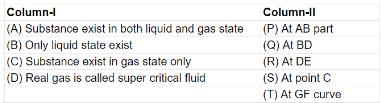Solution:

Refer to the following diagram and compare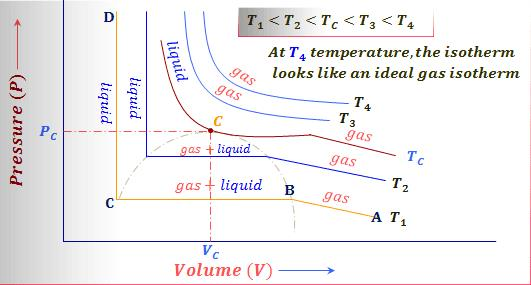QUESTION: 16

Match the Colum –I and Column-II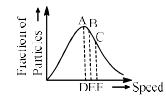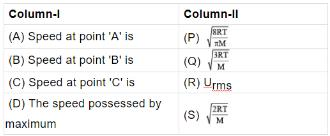Fraction of the gas particles

Solution:
QUESTION: 17

Initial temperature of an ideal gas is 75°C. At what temperature, the sample of neon gas would be heated to double its pressure, it the initial volume of gas is reduced by 15%:

Solution:
QUESTION: 18

At constant volume for a fixed number of moles of a gas, the pressure of the gas increases with the rise in temperature due to

Solution:
QUESTION: 19

Three flasks of equal volumes contain CH4, CO2, and Cl2 gases respectively. They will contain equal number of molecules if:

Solution:
QUESTION: 20

At 0°C and one atm pressure, a gas occupied 100 cc. If the pressure is increased to one and a halftime and temperature is increased by one-third of absolute temperature, then final volume of the gas will be:

Solution:

From the ideal gas equation,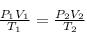Here,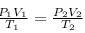are the initial and final volumes,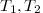are the initial and final temperatures and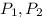are the initial and final pressure.

It is given in the problem that ,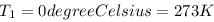Calculate the final temperature: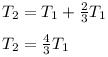Put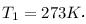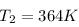Calculate the final volume of gas: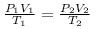Put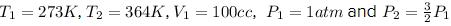in the above expression.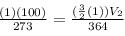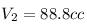Therefore, the final volume of gas is 88.8 cc.